# WEBfactory 2010

#### Log Conditions Section

Abstract

Log conditions are criteria to be met in order to record the signal values of the specified signals, defined by comparison to a constant or another signal value.

Log conditions are criteria to be met in order to record the signal values of the specified signals. The log conditions are defined by comparison to a constant or another signal value. The user can define logs and conditions for each server in Studio.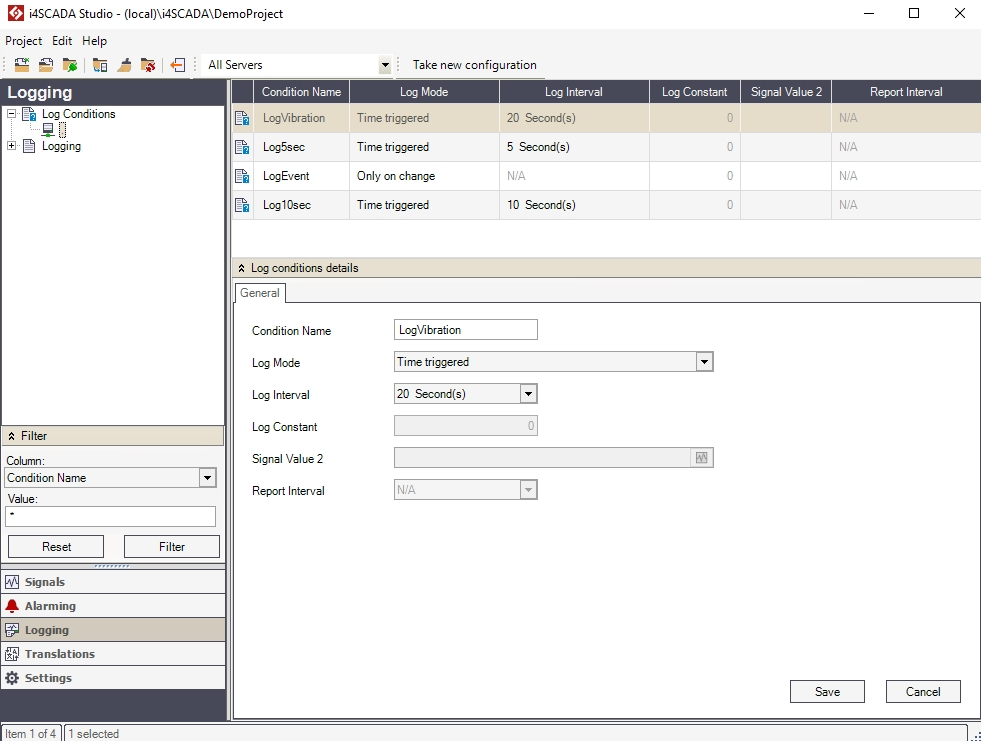The Log Conditions section

The main view displays the available log conditions in a grid form, having the following columns:

UI Option

Description

Condition Name

The name of the log condition.

Log Mode

The type of log condition. Can be:

• Time triggered: logs the signal value once per cycle (the cycle is defined by the Log Interval).

• Time triggered, when signal value > constant value: logs the signal value once per cycle (Log Interval) if the signal value is bigger than the constant value (Log Constant).

• Time triggered, when signal value < constant value: logs the signal value once per cycle (Log Interval) if the signal value is smaller than the constant value (Log Constant).

• Time triggered, when signal value > signal value 2: logs the signal value once per cycle (Log Interval) if the signal value is bigger than the value of another selected signal (Signal Value 2).

• Time triggered, when signal value < signal value 2: logs the signal value once per cycle (Log Interval) if the signal value is smaller than the value of another selected signal (Signal Value 2).

• Time triggered, when signal value = constant value: logs the signal value once per cycle (Log Interval) if the signal value is equal to the constant value (Log Constant).

• Time triggered, when signal value <> constant value: logs the signal value once per cycle (Log Interval) if the signal value is different from the constant value (Log Constant).

• Only on change: logs the signal value when it changes. The minimum time interval for logging is defined by the Update Time for each connector.

• Only on change, when signal value > constant value: logs the signal value when it changes if the signal value is bigger than the constant value (Log Constant).

• Only on change, when signal value < constant value: logs the signal value when it changes if the signal value is smaller than the constant value (Log Constant).

• Only on change, when signal value > signal value 2: logs the signal value when it changes if the signal value is bigger than the value of another selected signal (Signal Value 2).

• Only on change, when signal value < signal value 2: logs the signal value when it changes if the signal value is smaller than the value of another selected signal (Signal Value 2).

• Only on change, when signal value 2 = constant value: logs the signal value when it changes if the value of another selected signal (Signal Value 2) is equal to the constant value (Log Constant).

• Only on change, when signal value 2 <> constant value: logs the signal value when it changes if the value of another selected signal (Signal Value 2) is different from the constant value (Log Constant).

• Mean value: logs the average signal value once per cycle (Log Interval). The average is computed on a time span defined by the Report Interval.

• Counter mean value: logs the total average value once per cycle (Log Interval).

• Stored once, when the signal value 2 = constant value: logs the signal value once, when the value of another selected signal (Signal Value 2) is equal to the constant value (Log Constant).

• Stored once, when the signal value 2 <> constant value: logs the signal value once, when the value of another selected signal (Signal Value 2) is different from the constant value (Log Constant).

• Stored once, when the signal value 2 < constant value: logs the signal value once, when the value of another selected signal (Signal Value 2) is smaller than the constant value (Log Constant).

• Stored once, when the signal value 2 > constant value: logs the signal value once, when the value of another selected signal (Signal Value 2) is bigger than the constant value (Log Constant).

• Stored once, when the signal value 2 changes: logs the signal value once, when the value of another selected signal (Signal Value 2) changes.

The time interval between two consecutive logging operations. [N/A] means that there is no logging taking place.

Log Constant

A constant value used as a log condition parameter.

Signal Value 2

A secondary signal, not associated directly to the log condition, used for as a log condition parameter.

Report Interval

Defines the time span for calculating the average value. The average is obtained at the en of the time span defined by the Report Interval.

Right-clicking on the Log Conditions item in the Logging tree menu will open the contextual menu. The Log Conditions contextual menu provides a single option for creating new log conditions.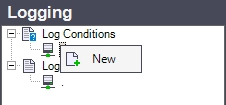UI Option

Description

New

Creates new a log condition.

Right-clicking on an log condition listed in the main panel will open the contextual menu, providing the options to edit the column in which the contextual menu is opened, copy, add or delete log conditions, expand or collapse the Log Condition Details panel and refresh the log conditions list.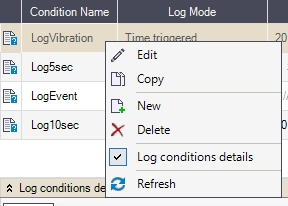Log Conditions contextual menu in the main panel

UI Option

Description

Edit

Edits the cell in which the contextual menu has been opened (where the user right-clicked).

Copy

Copies the selected log condition for the desired amount of times.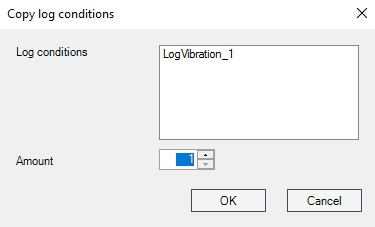New

Creates new log condition.

Delete

Deletes the selected log condition.

Log Condition Details

Expands/collapses the Log Condition Details panel.

Refresh

Reloads the list of log conditions.

The Log Condition Details panel is expanded by default when selecting the Log Conditions section in the Logging tree menu. It provides quick access to the selected log condition settings. This panel provides the same functionality as the main panel.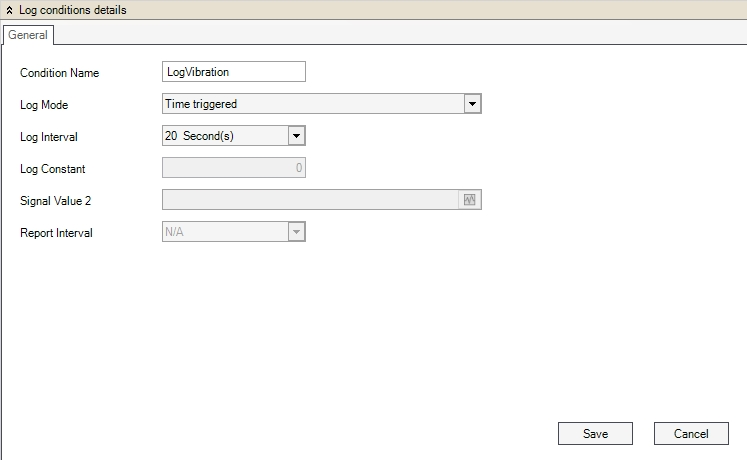Log Condition Details panel

UI Option

Description

Condition Name

The name of the log condition.

Log Mode

The type of log condition. Can be:

• Time triggered: logs the signal value once per cycle (the cycle is defined by the Log Interval).

• Time triggered, when signal value > constant value: logs the signal value once per cycle (Log Interval) if the signal value is bigger than the constant value (Log Constant).

• Time triggered, when signal value < constant value: logs the signal value once per cycle (Log Interval) if the signal value is smaller than the constant value (Log Constant).

• Time triggered, when signal value > signal value 2: logs the signal value once per cycle (Log Interval) if the signal value is bigger than the value of another selected signal (Signal Value 2).

• Time triggered, when signal value < signal value 2: logs the signal value once per cycle (Log Interval) if the signal value is smaller than the value of another selected signal (Signal Value 2).

• Time triggered, when signal value = constant value: logs the signal value once per cycle (Log Interval) if the signal value is equal to the constant value (Log Constant).

• Time triggered, when signal value <> constant value: logs the signal value once per cycle (Log Interval) if the signal value is different from the constant value (Log Constant).

• Only on change: logs the signal value when it changes. The minimum time interval for logging is defined by the Update Time for each connector.

• Only on change, when signal value > constant value: logs the signal value when it changes if the signal value is bigger than the constant value (Log Constant).

• Only on change, when signal value < constant value: logs the signal value when it changes if the signal value is smaller than the constant value (Log Constant).

• Only on change, when signal value > signal value 2: logs the signal value when it changes if the signal value is bigger than the value of another selected signal (Signal Value 2).

• Only on change, when signal value < signal value 2: logs the signal value when it changes if the signal value is smaller than the value of another selected signal (Signal Value 2).

• Only on change, when signal value 2 = constant value: logs the signal value when it changes if the value of another selected signal (Signal Value 2) is equal to the constant value (Log Constant).

• Only on change, when signal value 2 <> constant value: logs the signal value when it changes if the value of another selected signal (Signal Value 2) is different from the constant value (Log Constant).

• Mean value: logs the average signal value once per cycle (Log Interval). The average is computed on a time span defined by the Report Interval.

• Counter mean value: logs the total average value once per cycle (Log Interval).

• Stored once, when the signal value 2 = constant value: logs the signal value once, when the value of another selected signal (Signal Value 2) is equal to the constant value (Log Constant).

• Stored once, when the signal value 2 <> constant value: logs the signal value once, when the value of another selected signal (Signal Value 2) is different from the constant value (Log Constant).

• Stored once, when the signal value 2 < constant value: logs the signal value once, when the value of another selected signal (Signal Value 2) is smaller than the constant value (Log Constant).

• Stored once, when the signal value 2 > constant value: logs the signal value once, when the value of another selected signal (Signal Value 2) is bigger than the constant value (Log Constant).

• Stored once, when the signal value 2 changes: logs the signal value once, when the value of another selected signal (Signal Value 2) changes.